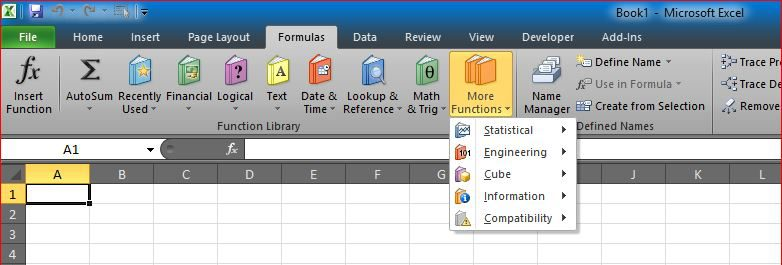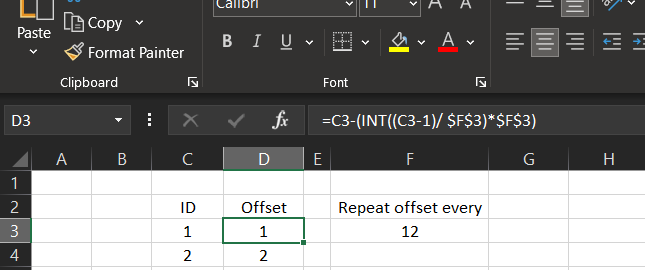# Repeated OffsetI needed to have an offset of columns to be repeated every 12 months.

This is part of a project, we needed to calculate the coefficient of sales prediction based on the month number, we do have coefficient saved in another table that repeated every year (12 months).

So we needed a formula to calculate the offset to multiply times coefficient to find the sales predictions.

I extracted a formula that allows me to reuse when I need a repeated offset to be calculated.

`=C3-(INT((C3-1)/ \$F\$3)*\$F\$3)`

The example here is for this pure offset repeater formula, once you change “Repeat offset every” in cell F3, you can see formulas in column D getting updated to reach that repeating pattern that we need.

This is the big formula in case interested (in R1C1 syntax)

The coefficient area starts in Column BS (R1C70) in sheet called D, while sales is in RC24 or RC25, RC26 has month number 1 through 12, RC29 has the row number needed from D sheet.

`=IfError(If( RC25=0, RC24, RC25 ) * Offset( 'D'!R1C70, RC29 - 1, RC26 + Column() - 30 -(INT((RC26 + Column() - 30 -1)/12)*12) ), 0) `